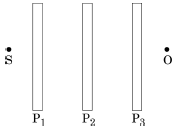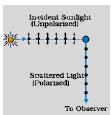# NEET Physics Wave Optics Questions Solved

Watch Physics > Wave Optics VideosBOARD

(a) Why does unpolarised light from a source show a variation in intensity when viewed through a polaroid which is rotated? Show with the help of a diagram, how unpolarised light from sun gets linearly polarised by scattering.

(b) Three identical polaroid sheets  are oriented so that the pass axis of ${\mathrm{P}}_{2}$ and ${\mathrm{P}}_{3}$ are inclined at angles $60°$ and $90°$ respectively with the pass axis of ${\mathrm{P}}_{1}$.

A monochromatic source S of unpolarized light of intensity ${\mathrm{I}}_{0}$ is kept in front of the polaroid sheet ${\mathrm{P}}_{1}$ as shown in the figure.

Determine the intensities of light as observed by the observer at O, when Polaroid ${\mathrm{P}}_{3}$ is rotated with respect to ${\mathrm{P}}_{2}$ at angles $\mathrm{\theta }=30°$ and $60°$(a) As per Malu's law

Transmitted intensity $\mathrm{I}={\mathrm{I}}_{0}{\mathrm{cos}}^{2}\mathrm{\theta }$

$\therefore$ The transmitted intensity will show a variation as per ${\mathrm{cos}}^{2}\mathrm{\theta }$             (1/2) marksThe electric field,of the incident wave, makes the electrons of the air molecules, acquire both components of motion ($↕$ as well as.)                                                    (1/2) marks

Charges accelerating parallel to $↕$, do not radiate energy towards the observer.

Hence the radiation , scattered towards the observer gets linearly polarised.

(b) We have, as per Malu's law

$\mathrm{I}={\mathrm{I}}_{0}{\mathrm{cos}}^{2}\mathrm{\theta }$                                                             (1/2) marks

$\therefore$ If the intensity of light, incident on ${\mathrm{P}}_{1}$ is ${\mathrm{I}}_{0}$, we have

${\mathrm{I}}_{1}$= Intensity transmitted through ${\mathrm{P}}_{1}$=$\frac{{\mathrm{I}}_{0}}{2}$

${\mathrm{I}}_{2}$= Intensity transmitted through ${\mathrm{P}}_{2}$=$\left(\frac{{\mathrm{I}}_{0}}{2}\right){\mathrm{cos}}^{2}60°=\frac{{I}_{0}}{8}$                  (1/2) marks

For $\mathrm{\theta }=30°$, we have

Angle between pass axis of ${\mathrm{P}}_{2}$ and ${\mathrm{P}}_{3}$

$\therefore {\mathrm{I}}_{3}$ can be either                                                        (1) marks

For $\mathrm{\theta }=60°$ , we have

Angle between pass axis of  ${\mathrm{P}}_{2}$ and ${\mathrm{P}}_{3}$

$\therefore {\mathrm{I}}_{3}$ can be either                                                          (1/2) marks

Difficulty Level:

• 93%
• 0%
Crack NEET with Online Course - Free Trial (Offer Valid Till September 21, 2019)